# Problem

Given a linked list, determine if it has a cycle in it.

Can you solve it without using extra space?

# Solution1: HashTable

Time complexity: O(n)

Space complexity: O(n)

# Solution2: Fast + Slow pointers

Time complexity: O(n)

Space complexity: O(1)

# Problem

https://leetcode.com/problems/find-eventual-safe-states/description/

In a directed graph, we start at some node and every turn, walk along a directed edge of the graph.  If we reach a node that is terminal (that is, it has no outgoing directed edges), we stop.

Now, say our starting node is eventually safe if and only if we must eventually walk to a terminal node.  More specifically, there exists a natural number K so that for any choice of where to walk, we must have stopped at a terminal node in less than K steps.

Which nodes are eventually safe?  Return them as an array in sorted order.

The directed graph has N nodes with labels 0, 1, ..., N-1, where N is the length of graph.  The graph is given in the following form: graph[i] is a list of labels j such that (i, j) is a directed edge of the graph.

Example:
Input: graph = [[1,2],[2,3],,,,[],[]]
Output: [2,4,5,6]
Here is a diagram of the above graph.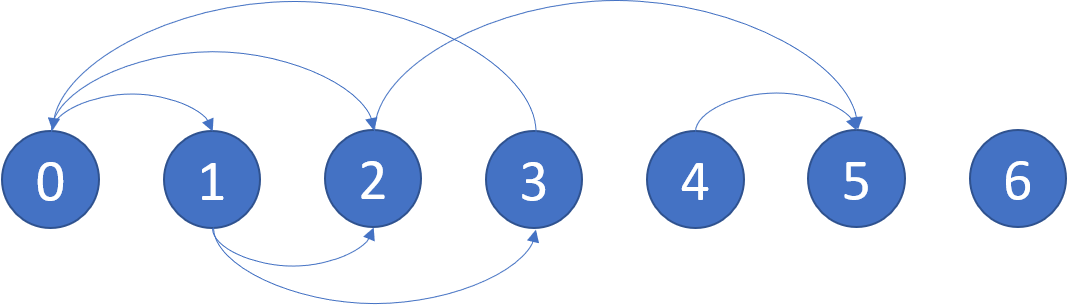Note:

• graph will have length at most 10000.
• The number of edges in the graph will not exceed 32000.
• Each graph[i] will be a sorted list of different integers, chosen within the range [0, graph.length - 1].

# Idea: Finding Cycles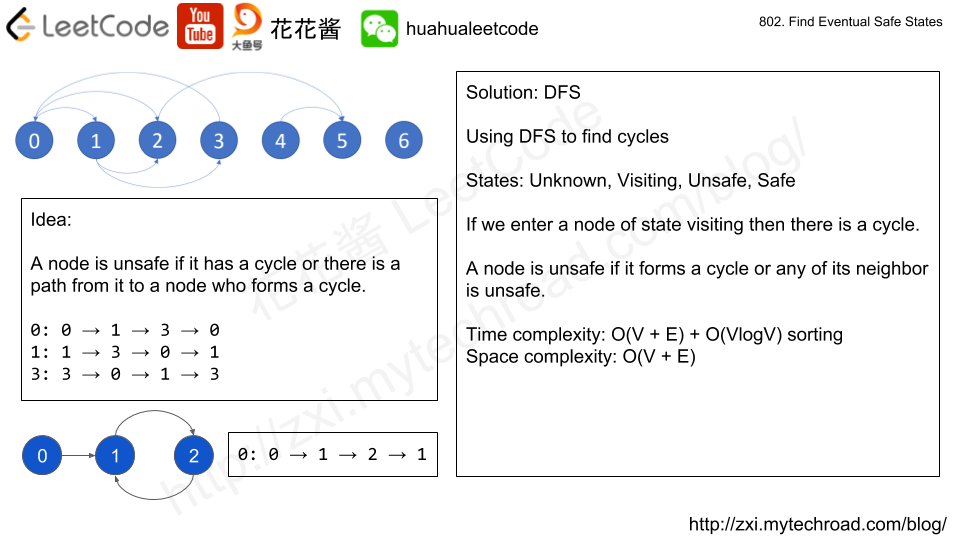# Solution 1: DFS

A node is safe if and only if: itself and all of its neighbors do not have any cycles.

Time complexity: O(V + E)

Space complexity: O(V + E)

# Problem

There are a total of n courses you have to take, labeled from 0 to n - 1.

Some courses may have prerequisites, for example to take course 0 you have to first take course 1, which is expressed as a pair: [0,1]

Given the total number of courses and a list of prerequisite pairs, return the ordering of courses you should take to finish all courses.

There may be multiple correct orders, you just need to return one of them. If it is impossible to finish all courses, return an empty array.

For example:

There are a total of 2 courses to take. To take course 1 you should have finished course 0. So the correct course order is [0,1]

There are a total of 4 courses to take. To take course 3 you should have finished both courses 1 and 2. Both courses 1 and 2 should be taken after you finished course 0. So one correct course order is [0,1,2,3]. Another correct ordering is[0,2,1,3].

Note:

1. The input prerequisites is a graph represented by a list of edges, not adjacency matrices. Read more about how a graph is represented.
2. You may assume that there are no duplicate edges in the input prerequisites.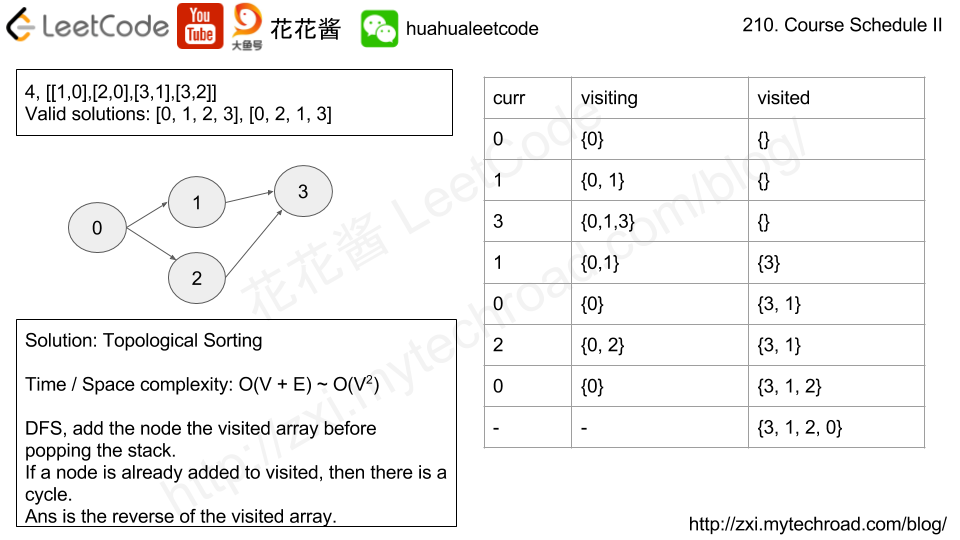# Idea

Topological sorting

# Solution 1: Topological Sorting

Time complexity: O(V+E)

Space complexity: O(V+E)

# Related Problems:

Problem:

There are a total of n courses you have to take, labeled from 0 to n - 1.

Some courses may have prerequisites, for example to take course 0 you have to first take course 1, which is expressed as a pair: [0,1]

Given the total number of courses and a list of prerequisite pairs, is it possible for you to finish all courses?

For example:

There are a total of 2 courses to take. To take course 1 you should have finished course 0. So it is possible.

There are a total of 2 courses to take. To take course 1 you should have finished course 0, and to take course 0 you should also have finished course 1. So it is impossible.

Idea:

Finding cycles O(n^2) -> Topological sort O(n)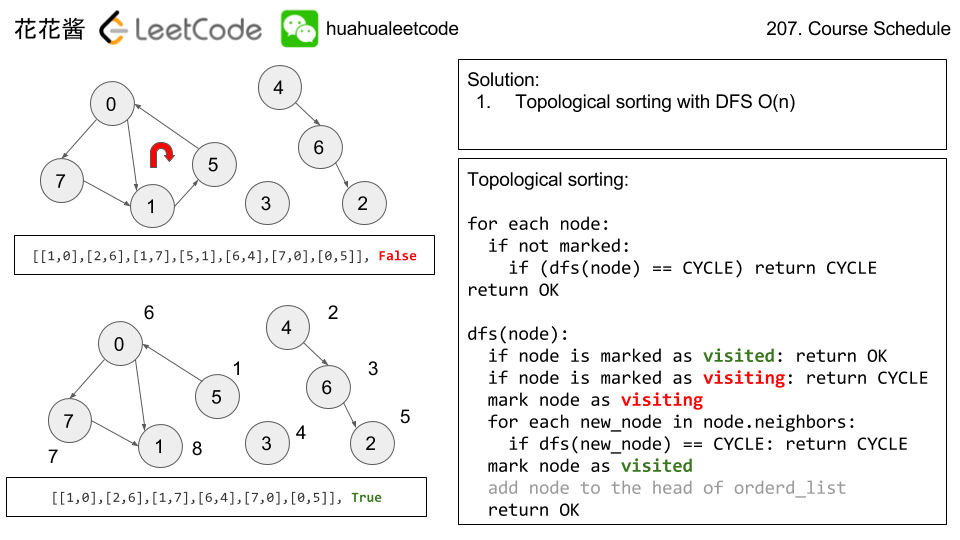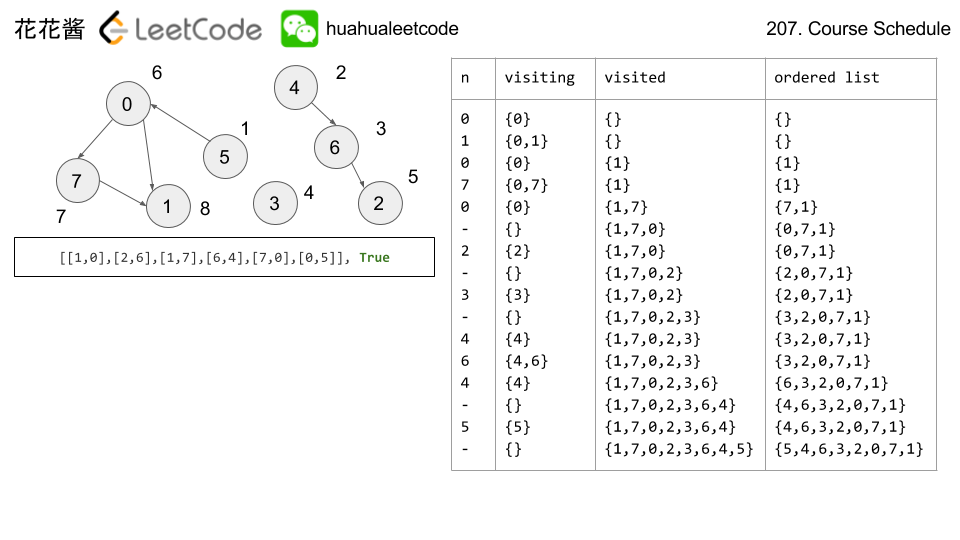# Solution 2: DFS Finding cycles

Time complexity: O(n^2)

Space complexity: O(n)

# Related Pr0blems:

Problem:

In this problem, a rooted tree is a directed graph such that, there is exactly one node (the root) for which all other nodes are descendants of this node, plus every node has exactly one parent, except for the root node which has no parents.

The given input is a directed graph that started as a rooted tree with N nodes (with distinct values 1, 2, …, N), with one additional directed edge added. The added edge has two different vertices chosen from 1 to N, and was not an edge that already existed.

The resulting graph is given as a 2D-array of edges. Each element of edges is a pair [u, v] that represents a directed edge connecting nodes u and v, where u is a parent of child v.

Return an edge that can be removed so that the resulting graph is a rooted tree of N nodes. If there are multiple answers, return the answer that occurs last in the given 2D-array.

Example 1:

Example 2:

Note:

• The size of the input 2D-array will be between 3 and 1000.
• Every integer represented in the 2D-array will be between 1 and N, where N is the size of the input array.

Idea:

Union Find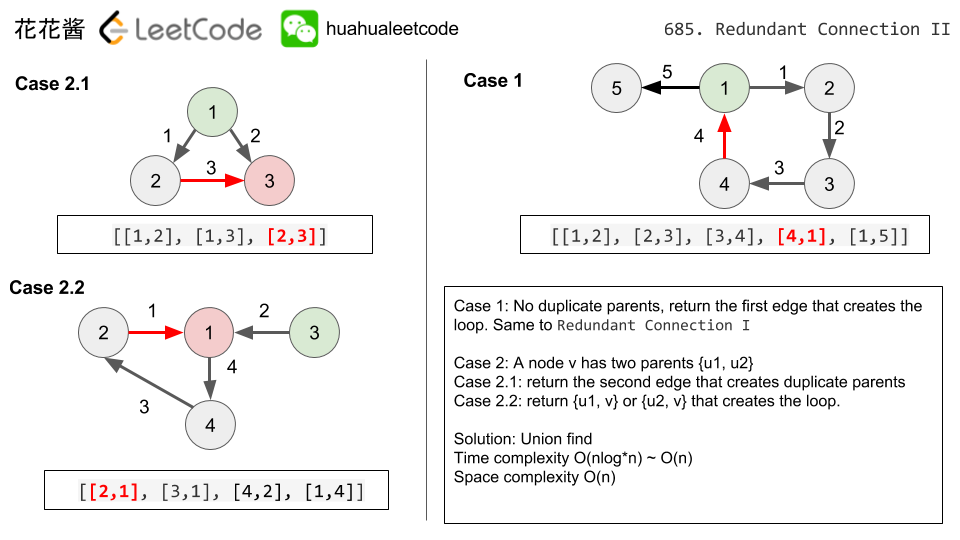Time complexity: O(nlog*n) ~ O(n)

Space complexity: O(n)

Solution:

C++

C++ / without using Union find

Python

Mission News Theme by Compete Themes.